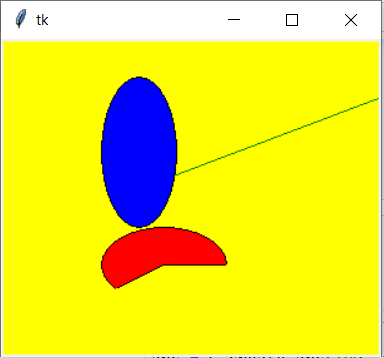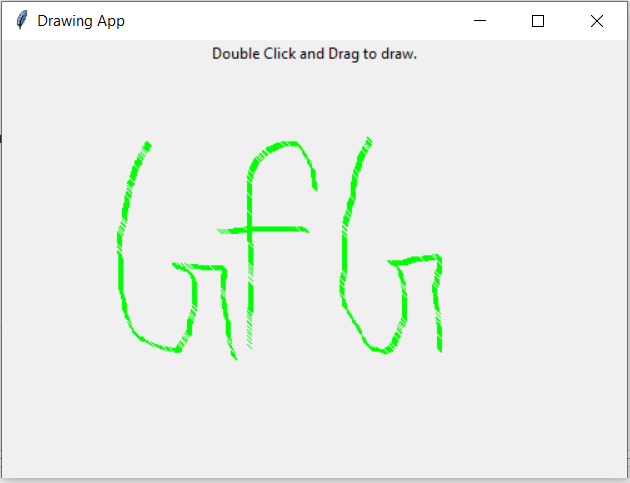Open in App
Not now

# Python Tkinter – Canvas Widget

• Difficulty Level : Medium
• Last Updated : 31 Aug, 2021

Tkinter is a GUI toolkit used in python to make user-friendly GUIs.Tkinter is the most commonly used and the most basic GUI framework available in python. Tkinter uses an object-oriented approach to make GUIs.

## Canvas widget

The Canvas widget lets us display various graphics on the application. It can be used to draw simple shapes to complicated graphs. We can also display various kinds of custom widgets according to our needs.

Syntax:

`C = Canvas(root, height, width, bd, bg, ..)`

Optional parameters:

• root = root window.
• height = height of the canvas widget.
• width = width of the canvas widget.
• bg = background colour for canvas.
• bd = border of the canvas window.
• scrollregion (w, n, e, s)tuple defined as a region for scrolling left, top, bottom and right.
• highlightcolor colour shown in the focus highlight.
• cursor It can be defined as a cursor for the canvas which can be a circle, a do, an arrow etc.
• confine decides if canvas can be accessed outside the scroll region.
• relief type of the border which can be SUNKEN, RAISED, GROOVE and RIDGE.

### Some common drawing methods:

• Creating an Oval
` oval = C.create_oval(x0, y0, x1, y1, options)`
• Creating an arc
` arc = C.create_arc(20, 50, 190, 240, start=0, extent=110, fill="red")`
• Creating a Line
` line = C.create_line(x0, y0, x1, y1, ..., xn, yn, options)`
• Creating a polygon
` oval = C.create_polygon(x0, y0, x1, y1, ...xn, yn, options)`

Example 1: Simple Shapes Drawing

## Python3

 `from` `tkinter ``import` `*`     `root ``=` `Tk()`   `C ``=` `Canvas(root, bg``=``"yellow"``,` `           ``height``=``250``, width``=``300``)`   `line ``=` `C.create_line(``108``, ``120``,` `                     ``320``, ``40``,` `                     ``fill``=``"green"``)`   `arc ``=` `C.create_arc(``180``, ``150``, ``80``,` `                   ``210``, start``=``0``,` `                   ``extent``=``220``,` `                   ``fill``=``"red"``)`   `oval ``=` `C.create_oval(``80``, ``30``, ``140``,` `                     ``150``,` `                     ``fill``=``"blue"``)`   `C.pack()` `mainloop()`

Output:Example 2: Simple Paint App

## Python3

 `from` `tkinter ``import` `*`     `root ``=` `Tk()`   `# Create Title` `root.title(  ``"Paint App "``)`   `# specify size` `root.geometry(``"500x350"``)`   `# define function when  ` `# mouse double click is enabled` `def` `paint( event ):` `   `  `    ``# Co-ordinates.` `    ``x1, y1, x2, y2 ``=` `( event.x ``-` `3` `),( event.y ``-` `3` `), ( event.x ``+` `3` `),( event.y ``+` `3` `) ` `    `  `    ``# Colour` `    ``Colour ``=` `"#000fff000"` `    `  `    ``# specify type of display` `    ``w.create_line( x1, y1, x2, ` `                  ``y2, fill ``=` `Colour )`     `# create canvas widget.` `w ``=` `Canvas(root, width ``=` `400``, height ``=` `250``) `   `# call function when double ` `# click is enabled.` `w.bind( ``""``, paint )`   `# create label.` `l ``=` `Label( root, text ``=` `"Double Click and Drag to draw."` `)` `l.pack()` `w.pack()`   `mainloop()`

Output:My Personal Notes arrow_drop_up
Related Articles